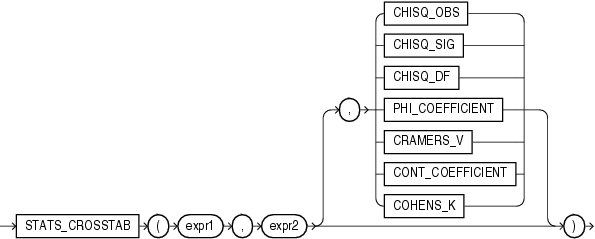# STATS_CROSSTAB

SyntaxDescription of the illustration stats_crosstab.gif

Purpose

Crosstabulation (commonly called crosstab) is a method used to analyze two nominal variables. The `STATS_CROSSTAB` function takes three arguments: two expressions and a return value of type `VARCHAR2`. `expr1` and `expr2` are the two variables being analyzed. The function returns one number, determined by the value of the third argument. If you omit the third argument, then the default is `CHISQ_SIG`. The meaning of the return values is shown in Table 5-4.

Table 5-4 STATS_CROSSTAB Return Values

Return Value Meaning

`CHISQ_OBS`

Observed value of chi-squared

`CHISQ_SIG`

Significance of observed chi-squared

`CHISQ_DF`

Degree of freedom for chi-squared

`PHI_COEFFICIENT`

Phi coefficient

`CRAMERS_V`

Cramer's V statistic

`CONT_COEFFICIENT`

Contingency coefficient

`COHENS_K`

Cohen's kappa

STATS_CROSSTAB Example The following example determines the strength of the association between gender and income level:

```SELECT STATS_CROSSTAB
(cust_gender, cust_income_level, 'CHISQ_OBS') chi_squared,
STATS_CROSSTAB
(cust_gender, cust_income_level, 'CHISQ_SIG') p_value,
STATS_CROSSTAB
(cust_gender, cust_income_level, 'PHI_COEFFICIENT') phi_coefficient
FROM sh.customers;

CHI_SQUARED    P_VALUE PHI_COEFFICIENT
----------- ---------- ---------------
251.690705 1.2364E-47      .067367056
```